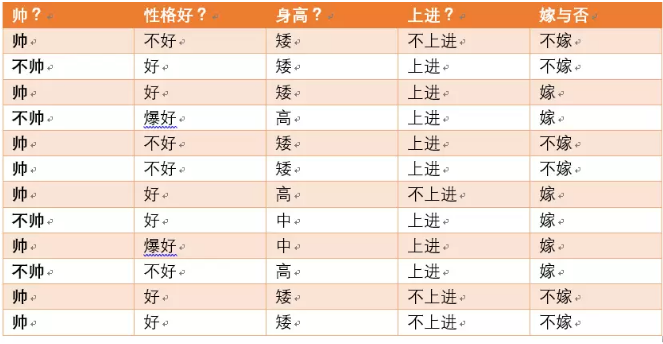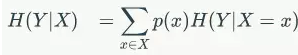﻿ 通俗理解决策树算法中的信息增益_51dev.com it技术开发者社区

# 通俗理解决策树算法中的信息增益

1  概念

2  例子H(Y|X = 矮) = -1/7log1/7-6/7log6/7=0.178

H(Y|X=中) = -1log1-0 = 0

H(Y|X=高） = -1log1-0=0

p(X = 矮) = 7/12,p(X =中) = 2/12,p(X=高) = 3/12

7/12*0.178+2/12*0+3/12*0 = 0.103

0.301-0.103=0.198

3  结论